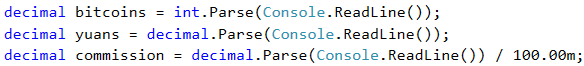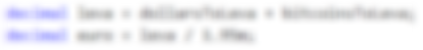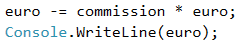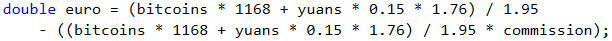# Problem: Money

Peter lives in Bulgaria and is keen about money exchange, trading, cryptocurrencies and financial markets. Some time ago, Peter bought Bitcoins (BTC) and paid for them in Bulgarian levs (BGN). Now, he is going on vacation in Europe and he needs Euro (EUR). Apart from the Bitcoins, he has Chinese yuans (CNY) as well. Peter wants to exchange his money for Euro for the tour. Write a program that calculates how much Euro he can buy, depending on the following exchange rates:

• 1 Bitcoin (BTC) = 1168 BGN
• 1 Chinese yuan (CNY) = 0.15 dollars (USD)
• 1 dollar (USD) = 1.76 BGN
• 1 Euro (EUR) = 1.95 BGN

The exchange office has commission fee within 0% to 5% from the final sum in Euro.

## Input Data

Three numbers are read from the console:

• On the first line – number of Bitcoins. Integer within the range of [0 … 20].
• On the second line – number of Chinese yuans. Floating-point number within the range of [0.00 … 50 000.00].
• On the third line – commission fee. Floating-point number within the range of [0.00 … 5.00].

## Output Data

Print one number on the console – the result of the exchange of currencies. Rounding is not necessary.

## Sample Input and Output

Input Output
1
5
5
569.668717948718

Explanation:

• 1 Bitcoin (BTC) = 1168 BGN
• 5 Chinese yuan (CNY) = 0.75 dollars
• 0.75 dollars (USD) = 1.32 BGN
• 1168 + 1.32 = 1169.32 BGN = 599.651282051282 Euro
• Commission fee: 5% of 599.651282051282 = 29.9825641025641
• Result: 599.651282051282 - 29.9825641025641 = 569.668717948718 Euro
Input Output Input Output
20
5678
2.4
12442.2442010256 7
50200.12
3
10659.4701177436

## Hints and Guidelines

Let's first think of the way we can solve the task again, before having started to write code.

### Idea for Solution

We see that the number of bitcoins and the number of Chinese yuans will be given in the input. The output should be in Euro. The exchange rates that we have to work with are specified in the task. We notice that we can only exchange the sum in BGN to EUR, therefore, we first have to calculate the whole sum that Peter has in BGN, and then calculate the output.

As we have information for the exchange rate of Bitcoins to BGN, we can directly exchange them. On the other hand, in order to get the value of Chinese yuans in BGN, first we have to exchange them in dollars, and then the dollars to BGN. Finally, we will sum the two values and calculate how much Euro that is.

Only the final step is left: calculating the commission fee and subtracting the new sum from the total one. We will obtain an integer for the commission fee, which will be a particular percent from the total sum. Let's divide it by 100, so as to calculate its percentage value and then multiply it by the sum in Euro. We will divide the result from the same sum and print the final sum on the console.

### Choosing Data Types

Bitcoins are given as an integer, therefore, we can declare a variable of int type for their value. For Chinese yuan and commission fee we obtain a floating-point number, therefore, we are going to use double. As double is the data type with bigger scope, and the output should also be a floating-point number, we will use it for the other variables we create as well.

### Solution

After we have built an idea on how to solve the task and we have chosen the data structures that we are going to use, it is time to get to writing the code. As in the previous tasks, we can divide the solution into three smaller tasks:

• Reading input from the console.
• Doing the calculations.
• Printing the output on the console.

We declare the variables that we are going to use and again we have to choose meaningful names, which are going to give us hints about the values they store. We initialize their values: with Console.ReadLine(…), we read the input numbers from the console and convert the string entered by the user to int or double.We do the necessary calculations:Finally, we calculate the commission fee value and subtract it from the sum in Euro. Let's pay attention to the way we could write this: Euro -= commission fee * Euro is the short way to write Euro = Euro - (commission fee * Euro). In this case, we use a combined assignment operator (-=) that subtracts the value of the operand to the right from the one to the left. The operator for multiplication (*) has a higher priority than the combined operator, this is why, the expression commission fee * Euro is performed first and then its value is divided.

The task does not specify special string formatting or rounding the result, therefore, we just have to calculate the output and print it on the console.Let's pay attention to something that applies to all other problems of this type: written like that, the solution of the task is pretty detailed. As the task itself is not too complex, in theory, we could write one big expression, where right after having taken the input, we calculate the output. For example, such expression would look like this:This code would print a correct result, but it is hard to read. It won't be easy to find out how it works and whether it contains any mistakes, as well as finding such and correcting them. Instead of one complex expression, it is better to write a few simpler ones and store their values in variables with appropriate names. This way, the code is cleaner and easily maintainable.

## Testing in the Judge System

Test your solution here: https://judge.softuni.org/Contests/Practice/Index/505#3.